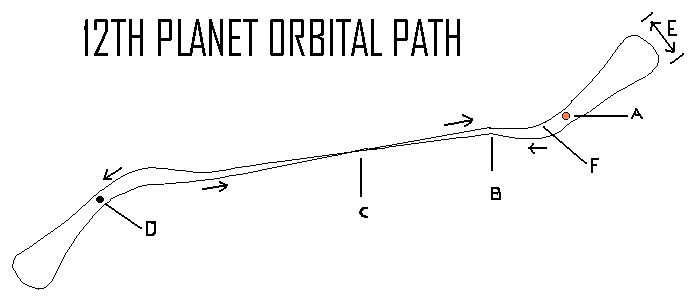The 12th Planet Orbital Diagram# Diagram Not Drawn to Scale

The Key:

A       The Sun

B       The Repulsion Point

C       The Cross Over or Dither Point

D       The Dark One

E       The Extreme Right End Swing of the Orbital Path

F       The Point Where the Orbital Path Reaches its Maximum Piercing Angle of 32 Degrees Through the Orbital Plane

Distance Key:

## A to D          18.724 S-P Units

### C to A          9.362   S-P Units

B to A          2.519   S-P Units

A to E          3.56     S-P Units

A unit of measure is equal to the mean distance between the Sun and the planet Pluto or S-P Units.  This is equal to 3.675 billion miles.

The angle between the Dark One and the orbital plane of the Sun’s solar system is -11 degrees.

Explanation of forces affecting the 12th Planet

Point of Reference:

Forces Acting on 12th Planet from Point A to E

Forces at Points B & C

Forces Acting on 12th Planet Between Point F and A